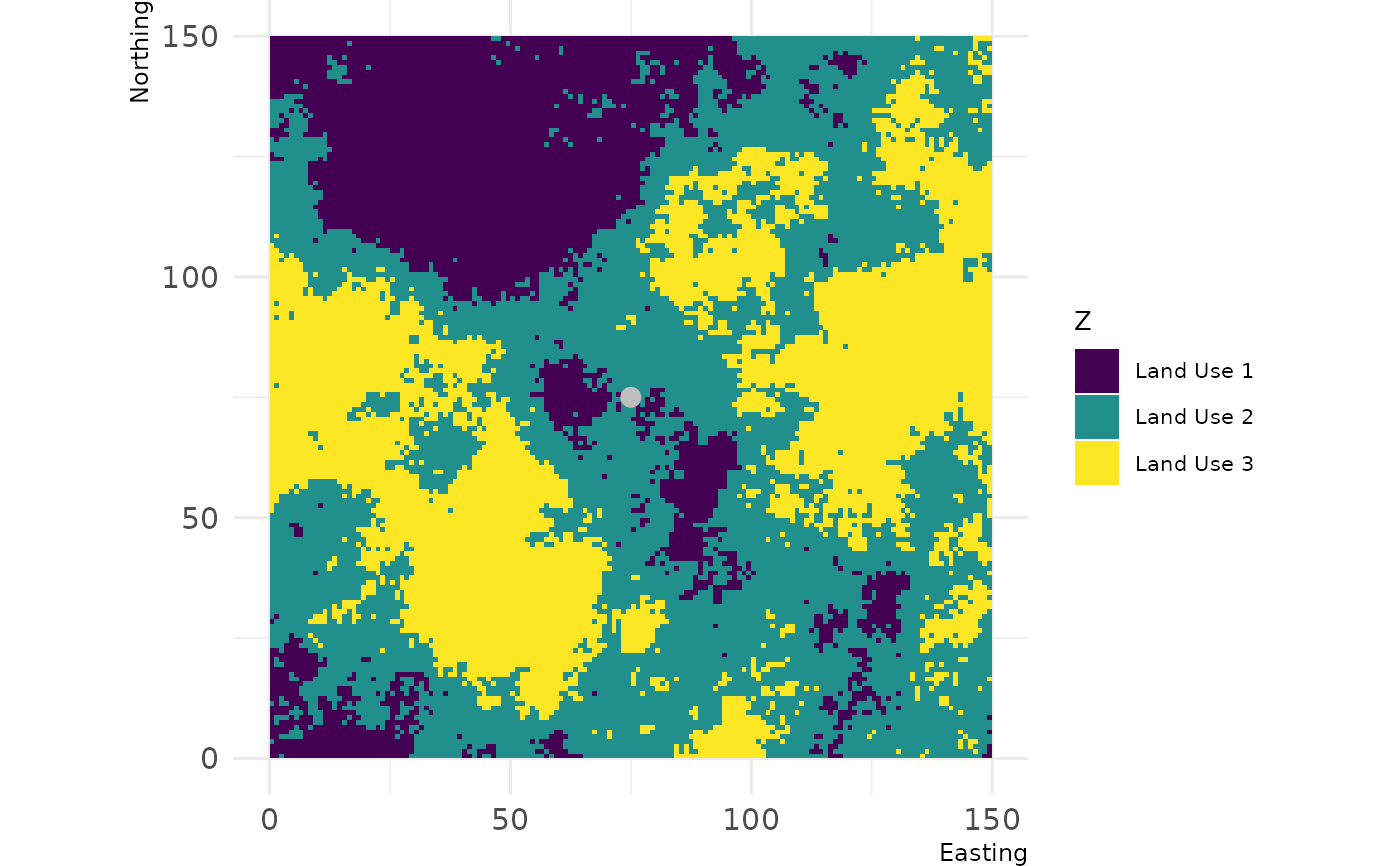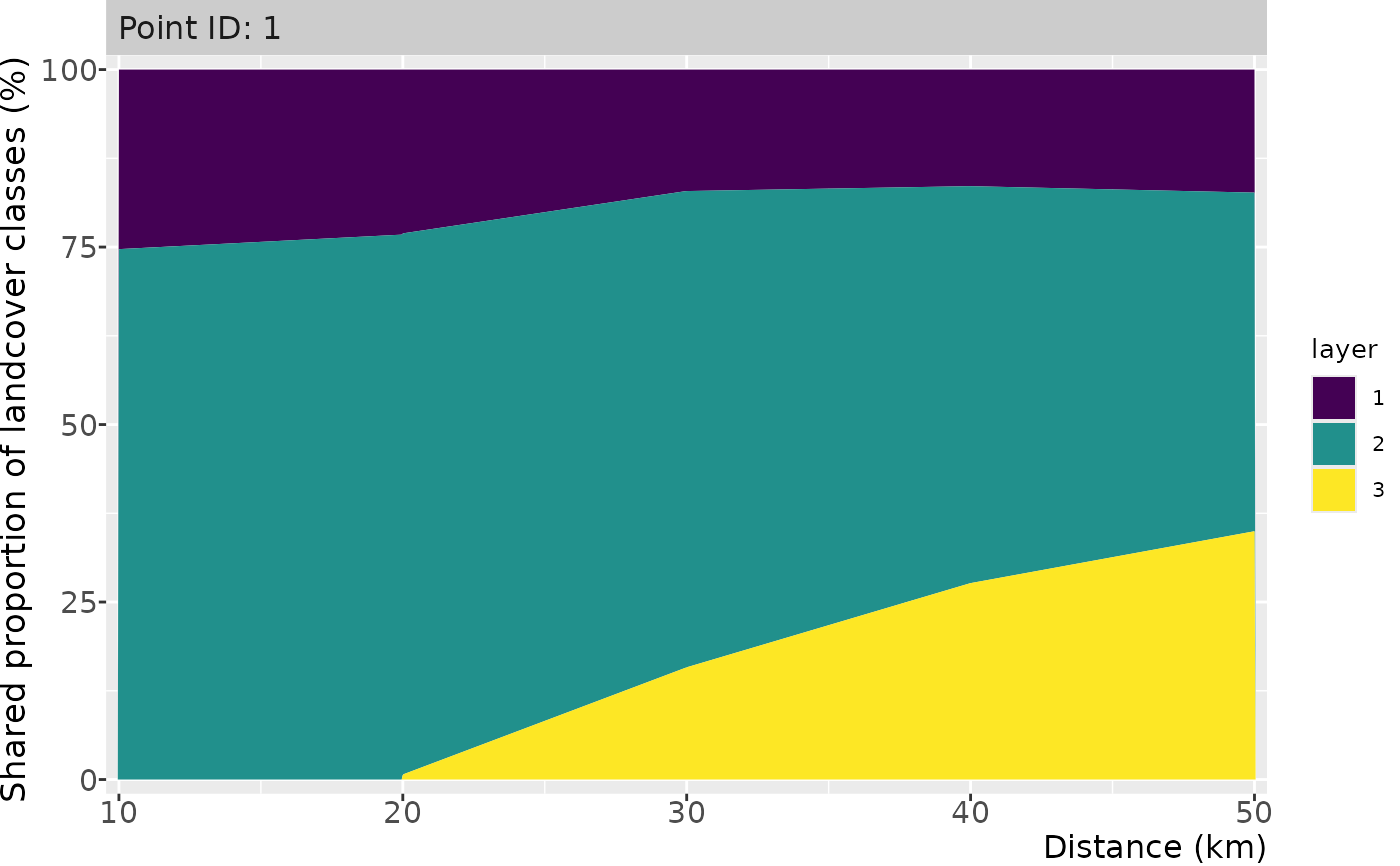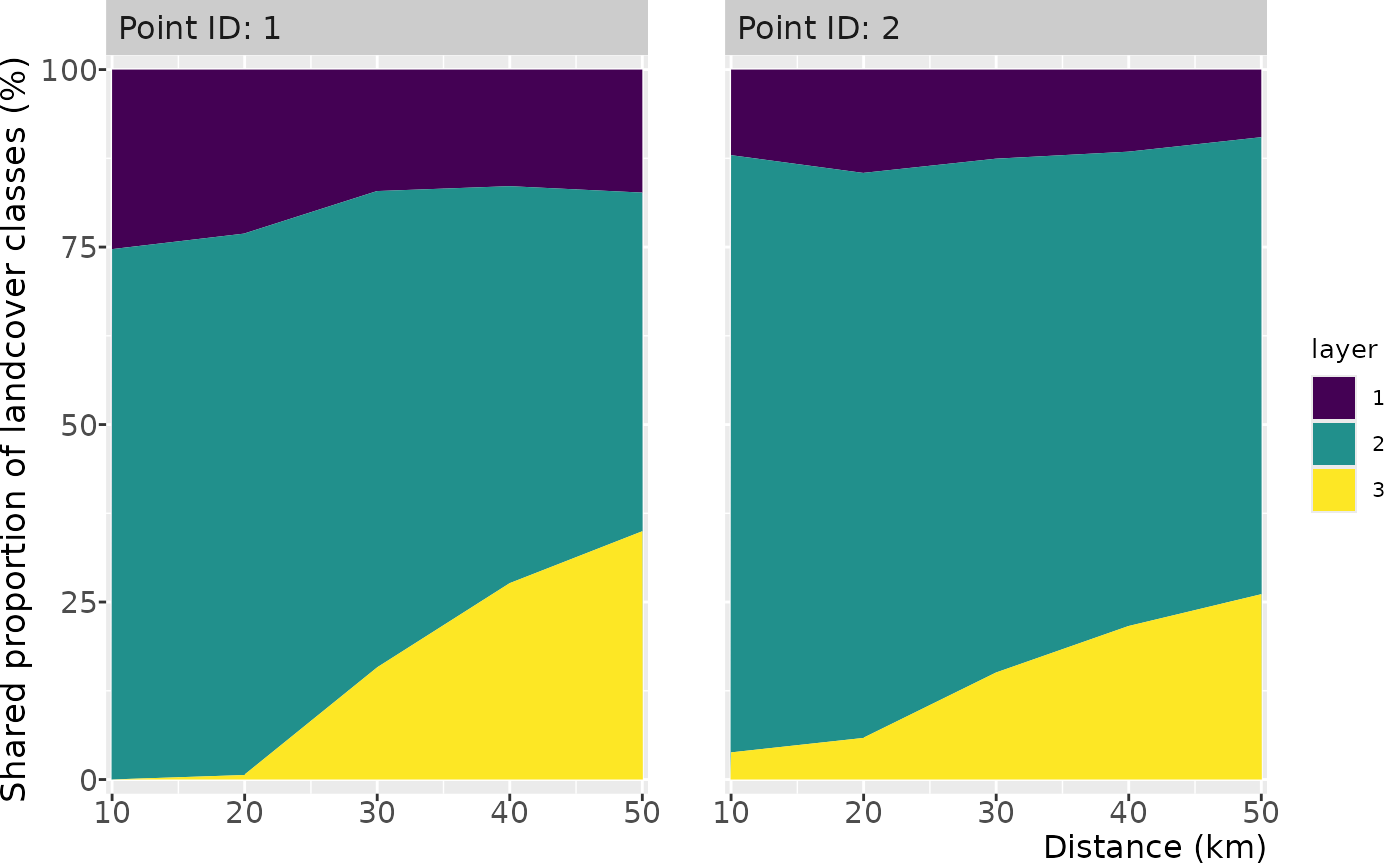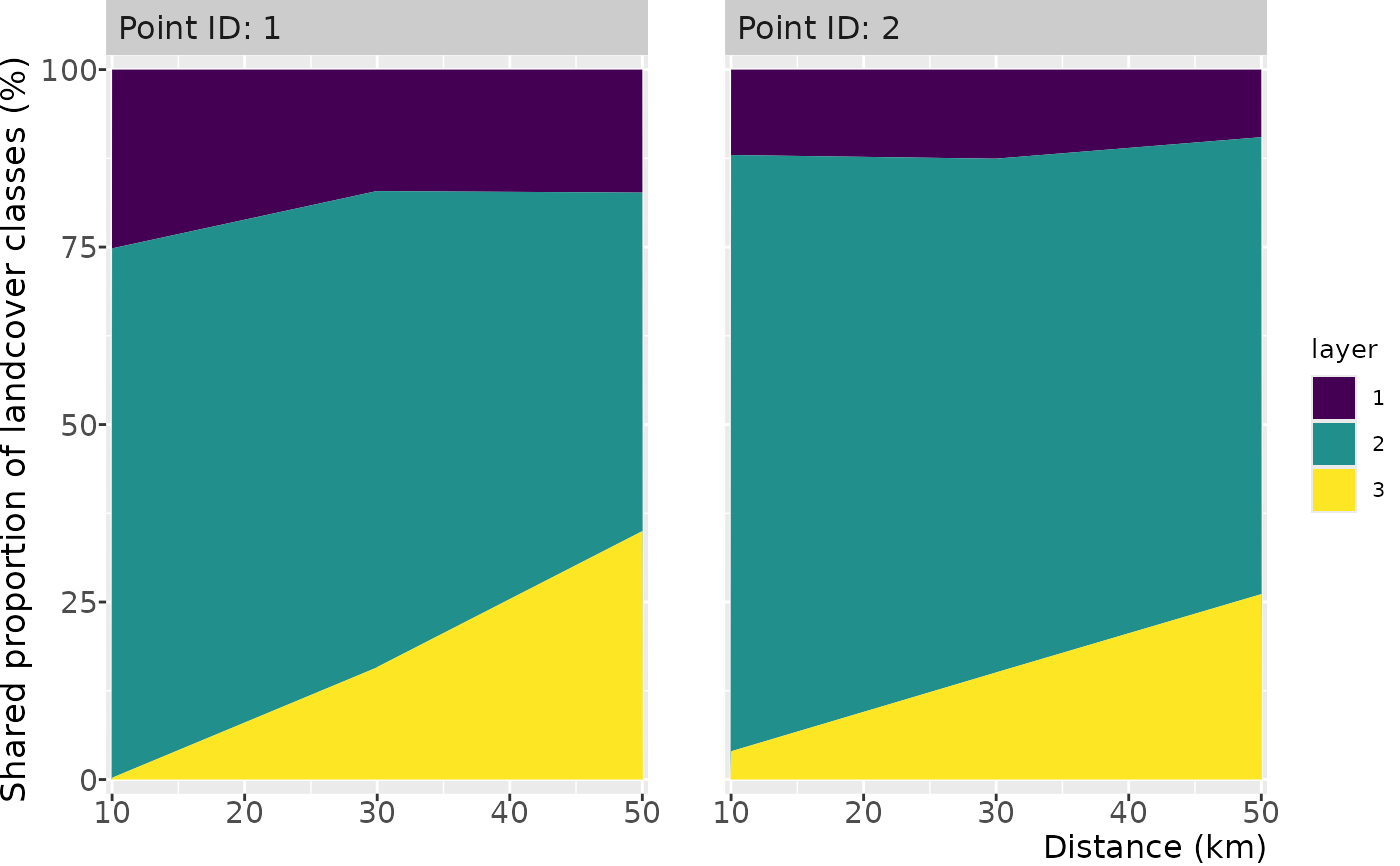Plot the landscape share in subsequential buffers around a/multiple point(s) of interest

## Usage

``````show_shareplot(
landscape,
points,
buffer_width,
max_width = NULL,
multibuffer_df = NULL,
return_df = FALSE
)

show_shareplot(
landscape,
points,
buffer_width,
max_width = NULL,
multibuffer_df = NULL,
return_df = FALSE
)``````

## Arguments

landscape

Raster* object

points

Point(s) represented by a two-column matrix or data.frame; SpatialPoints*; SpatialPolygons*; SpatialLines; Extent; a numeric vector representing cell numbers; or sf* POINT object

buffer_width

Buffer widths in which landscape share is measured. By default, it is a vector of buffer sizes, if `max_width = NULL`. If a value if provided for `max_width`, a series of buffer sizes is created, from `buffer_width` to `max_width`, with increases of `buffer_width`.

max_width

Max distance to which buffer_width is summed up; the x axis in the plot

multibuffer_df

`data.frame` with landscape share or a function from it already extracted, such as through the `util_extract_multibuffer()` function. If given, the other arguments (`landscape`, `points`, `buffer_width`, `max_width`) are ignored. Default is NULL.

return_df

Logical value indicating if a tibble with the underlying data should be returned

ggplot2 Object

## Examples

``````# create single point
new_point = matrix(c(75,75), ncol = 2)

# show landscape and point of interest
show_landscape(classified_landscape, discrete = TRUE) +
ggplot2::geom_point(data = data.frame(x = new_point[,1], y = new_point[,2]),
ggplot2::aes(x = x, y = y),
col = "grey", size = 3)# show single point share
show_shareplot(classified_landscape, new_point, 10, 50)# show multiple points share
new_points = matrix(c(75, 110, 75, 30), ncol = 2)
show_shareplot(classified_landscape, new_points, 10, 50)# irregular buffer widths
new_points = matrix(c(75, 110, 75, 30), ncol = 2)
show_shareplot(classified_landscape, new_points, c(10, 30, 50))# get data frame with results back
result <- show_shareplot(classified_landscape, new_points, 10, 50, return_df = TRUE)
result\$share_df
#> # A tibble: 30 × 4
#>    id          layer  freq buffer
#>    <chr>       <fct> <int>  <dbl>
#>  1 Point ID: 1 1        80     10
#>  2 Point ID: 2 1        38     10
#>  3 Point ID: 1 2       236     10
#>  4 Point ID: 2 2       266     10
#>  5 Point ID: 1 3         0     10
#>  6 Point ID: 2 3        12     10
#>  7 Point ID: 1 1       292     20
#>  8 Point ID: 2 1       184     20
#>  9 Point ID: 1 2       964     20
#> 10 Point ID: 2 2      1006     20
#> # … with 20 more rows

# use the output from util_extract_multibuffer
new_points = matrix(c(75, 110, 75, 30), ncol = 2)
df = util_extract_multibuffer(classified_landscape, new_points, 10, 50)
show_shareplot(multibuffer_df = df)``````